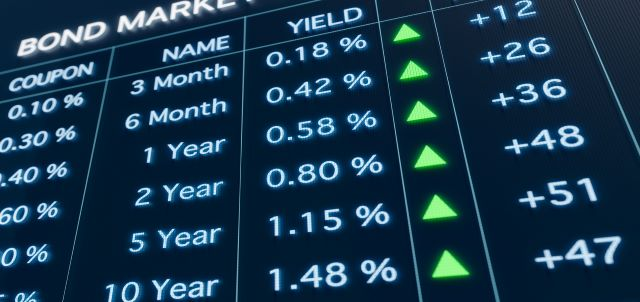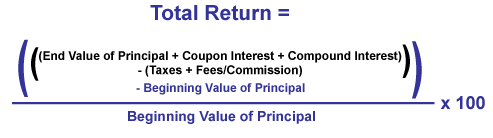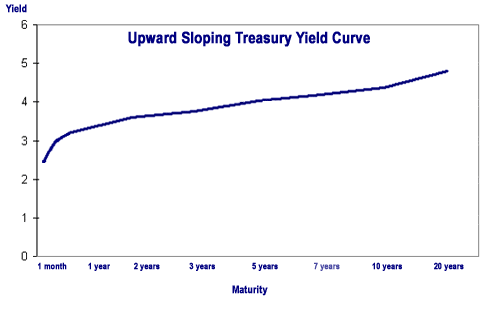Bonds

# Understanding Bond Yield and ReturnInvesting in bonds? You’ll want to know about yield and return.

Yield is a general term that relates to the return on the capital you invest in a bond. Price and yield are inversely related: As the price of a bond goes up, its yield goes down, and vice versa.

There are several definitions that are important to understand when talking about yield as it relates to bonds: coupon yield, current yield, yield-to-maturity, yield-to-call and yield-to-worst.

• Coupon yield, also known as the coupon rate, is the annual interest rate established when the bond is issued that does not change during the lifespan of the bond.
• Current yield is the bond's coupon yield divided by its current market price. If the current market price changes, the current yield will also change.

For example, if you buy a \$1,000 bond at par (often described as “trading at 100,” meaning 100 percent of its face value) and receive \$45 in annual interest payments, your coupon yield is 4.5 percent. If the price goes up and the bond subsequently trades at 103 (\$1,030), then the current yield will fall to 4.37 percent.

Current yield matters if you plan to sell your bond before maturity. But if you buy a new bond at par and hold it to maturity, your current yield when the bond matures will be the same as the coupon yield.

## Key Terms

Coupon and current yield only take you so far down the path of estimating the return your bond will deliver. For one, they don't measure the value of reinvested interest. They also aren't much help if your bond is called early—or if you want to evaluate the lowest yield you can receive from your bond. In these cases, you need to do some more advanced yield calculations. The following yields are worth knowing, and you can find them using FINRA’s Fixed Income Data.

Yield to maturity (YTM) is the overall interest rate earned by an investor who buys a bond at the market price and holds it until maturity. Mathematically, it is the discount rate at which the sum of all future cash flows (from coupons and principal repayment) equals the price of the bond. YTM is often quoted in terms of an annual rate and may differ from the bond’s coupon rate. It assumes that coupon and principal payments are made on time. It does not require dividends to be reinvested, but computations of YTM generally make that assumption. Further, it does not consider taxes paid by the investor or brokerage costs associated with the purchase.

Yield to call (YTC) is figured the same way as YTM, except instead of plugging in the number of months until a bond matures, you use a call date and the bond's call price. This calculation takes into account the impact on a bond's yield if it is called prior to maturity and should be performed using the first date on which the issuer could call the bond.

Yield to worst (YTW) is whichever of a bond's YTM and YTC is lower. If you want to know the most conservative potential return a bond can give you—and you should know it for every callable security—then perform this comparison.

Interest rates regularly fluctuate, making each reinvestment at the same rate virtually impossible. Thus, YTM and YTC are estimates only, and should be treated as such. While helpful, it's important to realize that YTM and YTC may not be the same as a bond's total return. Such a figure is only accurately computed when you sell a bond or when it matures.

## Figuring Bond Return

If you've held a bond over a long period of time, you might want to calculate its annual percent return, or the percent return divided by the number of years you've held the investment. For instance, a \$1,000 bond held over three years with a \$145 return has a 14.5 percent return, but a 4.83 percent annual return.

When you calculate your return, you should account for annual inflation. Calculating your real rate of return will give you an idea of the buying power your earnings will have in a given year. You can determine real return by subtracting the inflation rate from your percent return. As an example, an investment with 5 percent return during a year of 2 percent inflation is usually said to have a real return of 3 percent.

To figure total return, start with the value of the bond at maturity (or when you sold it) and add all of your coupon earnings and compounded interest. Subtract from this figure any taxes and any fees or commissions. Then subtract from this amount your original investment amount. This will give you the total amount of your total gain or loss on your bond investment. To figure the return as a percent, divide that number by the beginning value of your investment and multiply by 100: